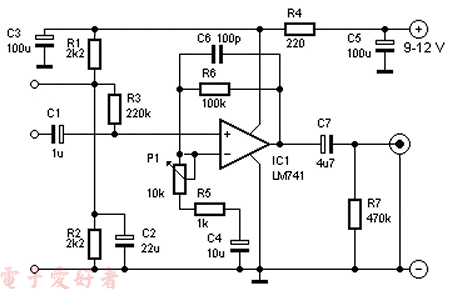# LM741麦克风前置放大器R1, R2 = 2,2 kΩ
R3 = 220 kΩ
R4 = 220 Ω
R5 = 1 kΩ
R6 = 100 kΩ
R7 = 470 kΩ
P1 = 10 kΩ
C1 = 1 µF/16 V
C2 = 22 µF/16 V
C3, C5 = 100 µF/16 V
C4 = 10 µF/16 V
C6 = 100 pF
C7 = 4,7 µF/16 V
IC1 = LM741